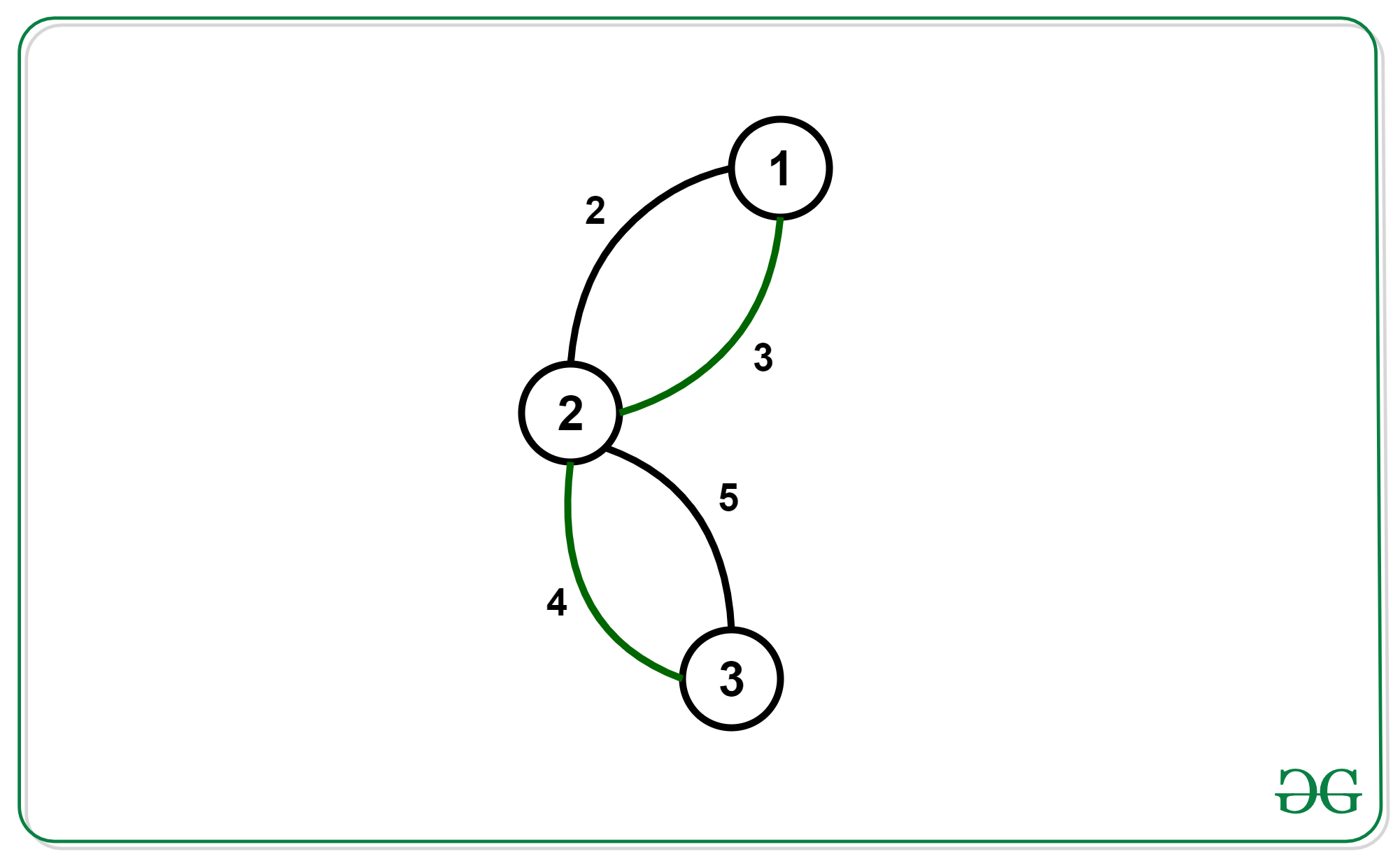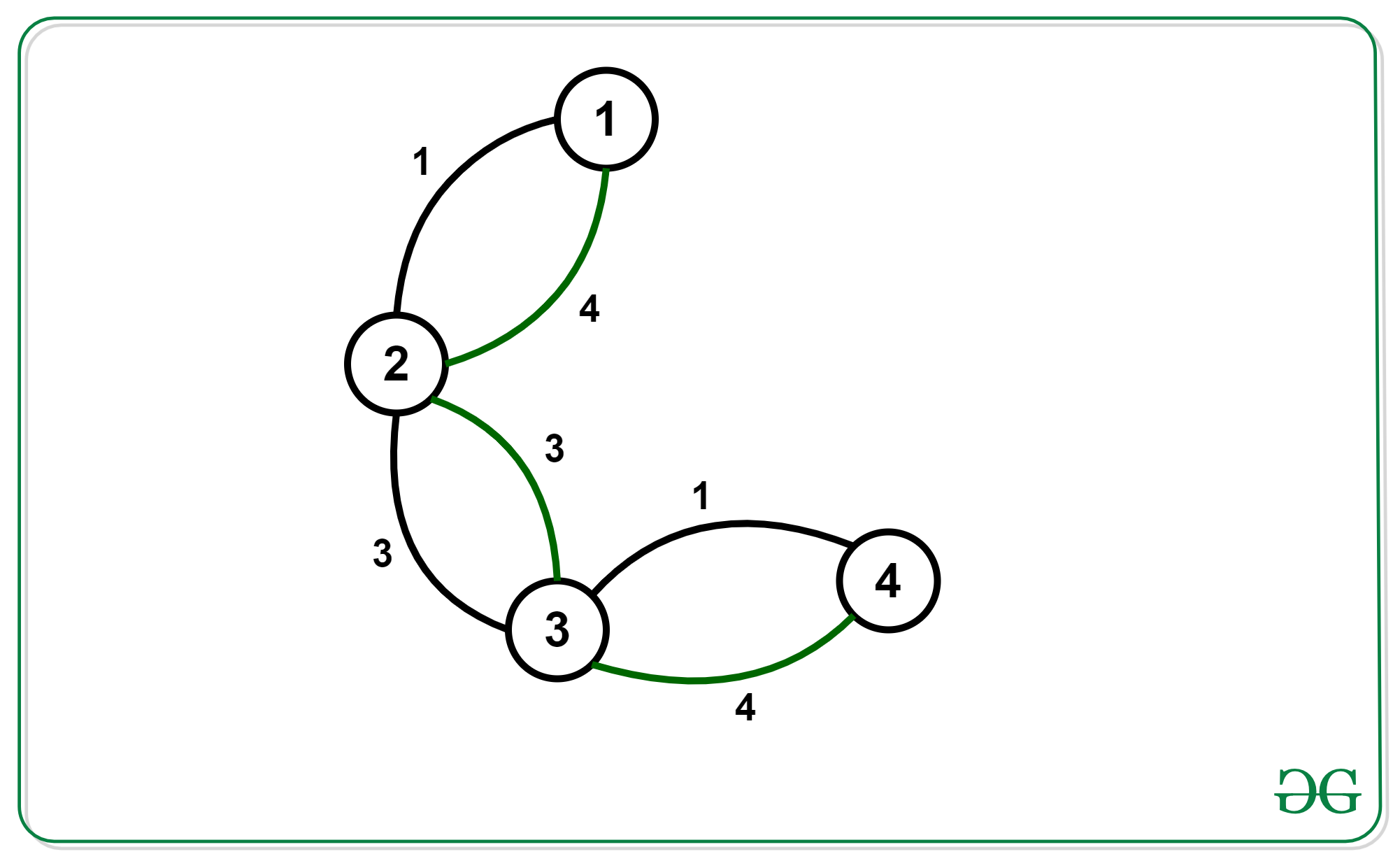# Find the minimum spanning tree with alternating colored edges

Given a graph with N nodes and M edges where each edge has a color (either black or green) and a cost associated with it. Find the minimum spanning tree of the graph such that every path in the tree is made up of alternating colored edges.

Examples:

Input: N = 3, M = 4Output: 6

Input: N = 4, M = 6Output: 4

## Recommended: Please try your approach on {IDE} first, before moving on to the solution.

Approach:

• The first observation we make here is every such kind of spanning tree will be a chain. To prove it, suppose we have a tree that is not a chain and every path in it is made up of alternating edges. Then we can deduce that at least 1 node has a degree of 3. Out of these 3 edges, at least 2 will have the same color. The path using these 2 edges will never follow the conditions and Hence, such kind of tree is always a chain.
• Now we can find a chain with minimum cost and alternating edges using bitmask-dp,
dp[mask(2^n)][Node(n)][col_of_last_edge(2)] where the mask is the bitmask of nodes we’ve added to the chain. Node is the last node we added to the chain.col_of_last_edge is the color of edge use to connect Node.
• To transition from 1 state to another state we visit the adjacency list of the last node and use those edges which have color != col_of_last_edge.

Below is the implementation of the above approach:

## C++

 `// C++ program for the ` `// above approach ` `#include ` `using` `namespace` `std; ` ` `  `int` `graph; ` ` `  `// Initializing dp of size = ` `// (2^18)*18*2. ` `long` `long` `dp[1 << 18]; ` ` `  `// Recursive Function to calculate ` `// Minimum Cost with alternate  ` `// colour edges ` `long` `long` `minCost(``int` `n, ``int` `m, ``int` `mask, ``int` `prev, ``int` `col) ` `{ ` `    ``// Base case ` `    ``if` `(mask == ((1 << n) - 1)) { ` `        ``return` `0; ` `    ``} ` `    ``// If already calculated ` `    ``if` `(dp[mask][prev][col == 1] != 0) { ` `        ``return` `dp[mask][prev][col == 1]; ` `    ``} ` ` `  `    ``long` `long` `ans = 1e9; ` ` `  `    ``for` `(``int` `i = 0; i < n; i++) { ` `        ``for` `(``int` `j = 0; j < 2; j++) { ` `            ``// Masking previous edges ` `            ``// as explained in above formula. ` `            ``if` `(graph[prev][i][j] && !(mask & (1 << i))  ` `                ``&& (j != col)) { ` `                ``long` `long` `z = graph[prev][i][j] +  ` `                              ``minCost(n,m,mask|(1<, ` `                      ``pair<``int``,``char``>>>& vp,``int` `m){ ` ` `  `  ``for` `(``int` `i = 0; i < m; i++) { ` `    ``int` `a = vp[i].first.first - 1; ` `    ``int` `b = vp[i].first.second - 1; ` `    ``int` `cost = vp[i].second.first; ` `    ``char` `color = vp[i].second.second; ` `    ``graph[a][b][color == ``'W'``] = cost; ` `    ``graph[b][a][color == ``'W'``] = cost; ` `  ``} ` `} ` ` `  `// Function to getCost ` `// for the Minimum Spanning ` `// Tree Formed ` `int` `getCost(``int` `n,``int` `m){ ` `     `  `    ``// Assigning maximum ` `    ``// possible value. ` `    ``long` `long` `ans = 1e9; ` ` `  `    ``for` `(``int` `i = 0; i < n; i++) { ` `        ``ans = min(ans, minCost(n, m, 1 << i, i, 2)); ` `    ``} ` ` `  `    ``if` `(ans != 1e9) { ` `        ``return` `ans; ` `    ``} ` `    ``else` `{ ` `        ``return` `-1; ` `    ``} ` `} ` ` `  `// Driver code ` `int` `main() ` `{ ` `    ``int` `n = 3, m = 4; ` `    ``vector, pair<``int``, ``char``> > > vp = { ` `        ``{ { 1, 2 }, { 2, ``'B'` `} }, ` `        ``{ { 1, 2 }, { 3, ``'W'` `} }, ` `        ``{ { 2, 3 }, { 4, ``'W'` `} }, ` `        ``{ { 2, 3 }, { 5, ``'B'` `} } ` `    ``}; ` ` `  `    ``makeGraph(vp,m); ` `    ``cout << getCost(n,m) << ``'\n'``; ` `     `  `    ``return` `0; ` `} `

## Python3

 `# Python implementation of the approach ` ` `  `graph ``=` `[[[``0``, ``0``] ``for` `i ``in` `range``(``18``)] ``for` `j ``in` `range``(``18``)] ` ` `  `# Initializing dp of size = ` `# (2^18)*18*2. ` `dp ``=` `[[[``0``, ``0``] ``for` `i ``in` `range``(``18``)] ``for` `j ``in` `range``(``1` `<< ``15``)] ` ` `  `# Recursive Function to calculate ` `# Minimum Cost with alternate ` `# colour edges ` `def` `minCost(n: ``int``, m: ``int``, mask:  ` `            ``int``, prev: ``int``, col: ``int``) ``-``> ``int``: ` `    ``global` `dp ` ` `  `    ``# Base case ` `    ``if` `mask ``=``=` `((``1` `<< n) ``-` `1``): ` `        ``return` `0` ` `  `    ``# If already calculated ` `    ``if` `dp[mask][prev][col ``=``=` `1``] !``=` `0``: ` `        ``return` `dp[mask][prev][col ``=``=` `1``] ` ` `  `    ``ans ``=` `int``(``1e9``) ` ` `  `    ``for` `i ``in` `range``(n): ` `        ``for` `j ``in` `range``(``2``): ` ` `  `            ``# Masking previous edges ` `            ``# as explained in above formula. ` `            ``if` `graph[prev][i][j] ``and` `not` `(mask & (``1` `<< i)) \ ` `                ``and` `(j !``=` `col): ` `                ``z ``=` `graph[prev][i][j] ``+` `minCost(n, ` `                        ``m, mask | (``1` `<< i), i, j) ` `                ``ans ``=` `min``(z, ans) ` ` `  `    ``dp[mask][prev][col ``=``=` `1``] ``=` `ans ` `    ``return` `dp[mask][prev][col ``=``=` `1``] ` ` `  `# Function to Adjacency ` `# List Representation ` `# of a Graph ` `def` `makeGraph(vp: ``list``, m: ``int``): ` `    ``global` `graph ` `    ``for` `i ``in` `range``(m): ` `        ``a ``=` `vp[i][``0``][``0``] ``-` `1` `        ``b ``=` `vp[i][``0``][``1``] ``-` `1` `        ``cost ``=` `vp[i][``1``][``0``] ` `        ``color ``=` `vp[i][``1``][``1``] ` `        ``graph[a][b][color ``=``=` `'W'``] ``=` `cost ` `        ``graph[b][a][color ``=``=` `'W'``] ``=` `cost ` ` `  `# Function to getCost ` `# for the Minimum Spanning ` `# Tree Formed ` `def` `getCost(n: ``int``, m: ``int``) ``-``> ``int``: ` ` `  `    ``# Assigning maximum ` `    ``# possible value. ` `    ``ans ``=` `int``(``1e9``) ` `    ``for` `i ``in` `range``(n): ` `        ``ans ``=` `min``(ans, minCost(n, m, ``1` `<< i, i, ``2``)) ` ` `  `    ``if` `ans !``=` `int``(``1e9``): ` `        ``return` `ans ` `    ``else``: ` `        ``return` `-``1` ` `  `# Driver Code ` `if` `__name__ ``=``=` `"__main__"``: ` ` `  `    ``n ``=` `3` `    ``m ``=` `4` `    ``vp ``=` `[[[``1``, ``2``], [``2``, ``'B'``]], ` `        ``[[``1``, ``2``], [``3``, ``'W'``]], ` `        ``[[``2``, ``3``], [``4``, ``'W'``]], ` `        ``[[``2``, ``3``], [``5``, ``'B'``]]] ` `    ``makeGraph(vp, m) ` `    ``print``(getCost(n, m)) ` ` `  `# This code is contributed by ` `# sanjeev2552 `

Output:

```6
```

Time Complexity: O(2^N * (M + N))My Personal Notes arrow_drop_upCheck out this Author's contributed articles.

If you like GeeksforGeeks and would like to contribute, you can also write an article using contribute.geeksforgeeks.org or mail your article to contribute@geeksforgeeks.org. See your article appearing on the GeeksforGeeks main page and help other Geeks.

Please Improve this article if you find anything incorrect by clicking on the "Improve Article" button below.

Improved By : sanjeev2552